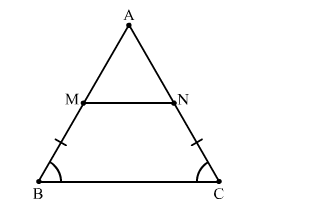# In △ABC, M and N are points on AB and ACQuestion:

In △ABC, M and N are points on AB and AC respectively such that BM = CN. If ∠B = ∠C then show that MN∥BC.

Solution:In △ABC, ∠B = ∠C
∴AB = AC (Sides opposite to equal angle are equal)
Subtracting BM from both sides, we get
AB − BM = AC − BM
⇒AB − BM = AC − CN           (∵BM = CN)
⇒AM = AN
∴∠AMN = ∠ANM (Angles opposite to equal sides are equal)
Now, In △ABC,
∠A + ∠B + ∠C = 180o                     .....(1)
(Angle Sum Property of triangle)
Again In In △AMN,
∠A +∠AMN + ∠ANM = 180o          ......(2)
(Angle Sum Property of triangle)
From (1) and (2), we get
∠B + ∠C = ∠AMN + ∠ANM
⇒2∠B  = 2∠AMN
⇒∠B  = ∠AMN
Since, ∠B  and ∠AMN are corresponding angles.
∴ MN∥BC